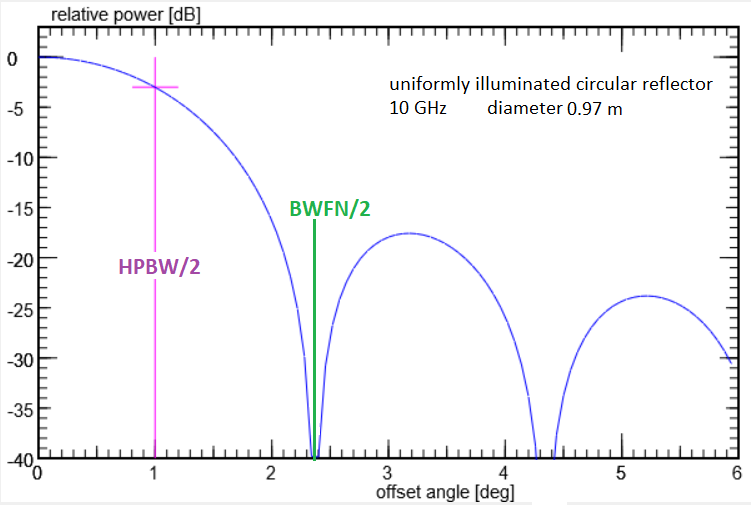AntennaCalculator

Joachim Köppen Kiel Jul.2016

This utility computes the properties of a uniformly illuminated circular dish antenna such as the gain, the half-power beam width (HPBW), and the beam width between first nulls (BWFN) of the main lobe from the parameters such as frequency, dish diameter, and aperture efficiency. Conversely, one can enter the measured HPBW and find the gain, effective diameter, and aperture efficiency for a given dish size. The formulae are correct as long as the HPBW is less than a few degrees. The characteristic antenna pattern is shown in the plot at right. The example given has a HPBW of 2°; multiply the abscissa value by HPBW/2 to get the actual offset angle.

Input fields which accept input values are marked by light green colour. A click on a white field makes it an input field. But some are always necessary for input, and others are output only.

Hit the Enter key to recompute with the new values.

It also computes the antenna temperature and signal to noise ratio Y = (S+N)/N of a celestial source with specified radio flux when observed by the antenna system with specified system temperature. Note that this gives correct values only if the source's angular diameter is smaller than the HPBW, so that all its flux is received!

Gain [dBi]
Gain [dBd]
Linear gain
Solid angle ΩA [sterad]
HPBW [deg]
BWFN [deg]

Frequency [MHz]
Wavelength [cm]
Aperture efficiency
effective Area [m²]
effective Diameter [m]
true Diameter [m]

Sensitivity [Jy/K]
Source flux [Jy]
Antenna temperature [K]
System temperature [K]
Y [dB]last update: Dec 2017 J.Köppen